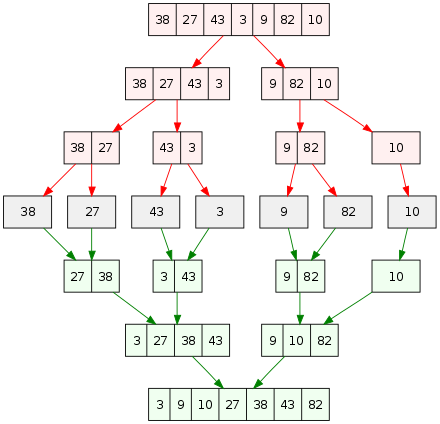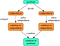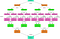# Diving into Divide and Conquer Algorithmsource : wikiwand.com

As a programmer, we constantly try to refine our code by improving its efficiency in terms of time taken or storage used.

Divide and conquer is an amazing technique for optimizing algorithms, whether we are trying to write efficient code or ace a competition. Its application lies in widely used algorithms such as binary search, merge sort, and quick sort.

Divide and Conquer breaks a problem into various sub-parts ,recursively solves them, and finally integrate the solutions of different sub-parts to solve the original problem. Since divide and conquer solves problems of the sub-parts recursively, each part must be smaller than the original problem.

The Divide and Conquer algorithm can be roughly divided into three parts:

1. Divide the problem into a number of sub-parts that are smaller instances of the same problem.

2. Conquer the sub-parts by solving their problem recursively.

3. Combine the solutions to the sub-parts to form the solution for the original problem.

The steps of a divide-and-conquer algorithm can be easily remembered as divide, conquer, combine. Here’s how we can view the working of the algorithm (assuming that each dividing step creates two sub-parts):source: Khanacademy.org

If two more steps in the recursive process are expanded, then:source : Khanacademy.org

# Application of Divide and Conquer algorithm :

Some standard algorithms that follow the rules and technique of the Divide and Conquer algorithm:

1. Binary Search is a type of searching algorithm in which at each step, the input element x is compared with the value of the middle element in the array. If x is greater than the middle element, then the algorithm solves for the right side of the middle element, else solves for the left side of the middle element.

2. Quicksort is a type of sorting algorithm where a pivot element is picked us and array elements are rearranged in such a way that all the elements smaller than the picked pivot moves to the left side and elements which are greater make their way to the left. Then in a recursive way, the algorithm sorts the subarrays on both the sides of the pivot element.

3. Merge Sort is also a type of sorting algorithm where the array is divided into two halves which are recursively sorted and then merged.

4. Closest Pair of Points The problem is to search for the closest pair of points among a set of points in the x-y plane. By calculating the distances of every pair of points and comparing them with each other to find the minimum, this problem can be solved in O(n²) time. Using the Divide and Conquer algorithm, the time complexity of the solution is O(nlogn).

5. Strassen’s Algorithm is an algorithm that efficiently multiplies two matrices. It needs 3 nested loops and has a time complexity of O(n³). Using Strassen’s algorithm, two matrices can be multiplied in O(n².8974) time.

6. Cooley–Tukey Fast Fourier Transform (FFT) algorithm is a common fast fourier transform algorithm. It uses the divide and conquer algorithm with a time complexity of O(nlogn).

Divide and Conquer algorithm:

`DAC(a, i, j){    if(small(a, i, j))      return(Solution(a, i, j))    else       m = divide(a, i, j)               // f1(n)      b = DAC(a, i, mid)                 // T(n/2)      c = DAC(a, mid+1, j)            // T(n/2)      d = combine(b, c)                 // f2(n)   return(d)}`

Recurrence Relation for Divide and Conquer algorithm :

This is recurrence relation for the above code snippet.

`O(1) if n is smallT(n) =     f1(n) + 2T(n/2) + f2(n)`

# Time Complexity :

The complexity of the divide and conquer algorithm: (using Master Theorem)

`T(n) = T(n/b) + f(n),where,n = size of inputa = number of subproblems in the recursionn/b = size of each subproblem. All subproblems are assumed to have the same size.f(n) = cost of the work done outside the recursive call, which includes the cost of dividing the problem and cost of merging the solutions`

# Advantages :

• Divide and Conquer is much faster than other algorithms and has helped successfully solve one of the biggest problems; The tower of Hanoi, which is a challenging and complicated mathematical puzzle.
• It uses cache memory to solve the problems of simple sub-parts instead of accessing the main memory which is slower comparatively. This makes the algorithm efficient in terms of space complexity.
• It is more proficient than its counterpart Brute Force technique.
• It inhibits parallelism and does not involve any modification . This algorithm is handled by systems which incorporates parallel processing.

# Disadvantages :

• There is a necessary requirement of high memory management as this algorithm is designed to incorporate recursion.
• Space might be overused by an explicit stack.
• There is a chance of system crashing if the algorithm performs recursion greater than the stack present in the CPU

Dynamic Programming vs Divide and Conquer

Both paradigms involve dividing the given problem into sub-parts and solving them individually . So how to decide on which one to choose among them for a given problem? When a same sub-part isn’t evaluated many time, one should opt for Divide and Conquer algorithm. Otherwise, memoisation or dynamic programming should be chosen.

For example, in binary search which is a Divide and Conquer algorithm, the problem of the same sub-part is never evaluated again. On the other hand, when we are calculating the nth term of a Fibonacci series, evaluation of the same part is required which makes the use of dynamic programming more favorable.

Divide and Conquer way of approach:

`fib(n)    If n < 2, return 1    Else , return f(n - 1) + f(n -2)`

Dynamic way of approach:

`a = []fib(n)    If n in a:         return a[n]     else,             If n < 2, f = 1        else , f = f(n - 1) + f(n -2)        a[n] = f        return f`

Check out my YouTube video for more information on Divide and Conquer algorithm:

https://www.youtube.com/watch?v=Nb4EpzHG0BI&t=4s

By

Anushka Garg

(As part of DAA project, E19CSE252)